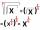Tank

In the middle of a cylindrical tank with a bottom diameter 251 cm is standing rod which is 13 cm above the water surface. If we bank rod its end reach surface of the water just by the tank wall.

How deep is the tank?

Result

h =  599.28 cm

Solution:Leave us a comment of example and its solution (i.e. if it is still somewhat unclear...):Be the first to comment!To solve this example are needed these knowledge from mathematics:

Looking for help with calculating roots of a quadratic equation? Pythagorean theorem is the base for the right triangle calculator.

Next similar examples:

1. Eq with reciprocalSolve given equation with reciprocal member: a-6/a+10=4/8
2. The chordCalculate a chord length which the distance from the center of the circle (S, 6 cm) equals 3 cm.Find the roots of the quadratic equation: 3x2-4x + (-4) = 0.Solve quadratic equation: 2x2-58x+396=0
5. EquationEquation ? has one root x1 = 8. Determine the coefficient b and the second root x2.
6. Square rootsWhat is equal to the product of the square roots of 295936?
7. ProductThe product of two consecutive odd numbers is 8463. What are this numbers?
8. Theorem proveWe want to prove the sentence: If the natural number n is divisible by six, then n is divisible by three. From what assumption we started?
9. CalculationHow much is sum of square root of six and the square root of 225?
10. RootsDetermine the quadratic equation absolute coefficient q, that the equation has a real double root and the root x calculate: ?
11. DiscriminantDetermine the discriminant of the equation: ?
12. EQ2Solve quadratic equation: ?
13. Chord 5It is given circle k / S; 5 cm /. Its chord MN is 3 cm away from the center of the circle . Calculate its length.
14. Completing squareSolve the quadratic equation: m2=4m+20 using completing the square method
15. FractionFor what x expression ? equals zero?
16. 6 termsFind the first six terms of the sequence. a1 = 7, an = an-1 + 6
17. TreesA certain species of tree grows an average of 0.5 cm per week. Write an equation for the sequence that represents the weekly height of this tree in centimeters if the measurements begin when the tree is 200 centimeters tall.